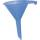# The funnel

The funnel has the shape of an equilateral cone. Calculate the content of the area wetted with water if you pour 3 liters of water into the funnel.

S =  8.7876 dm2

### Step-by-step explanation:Did you find an error or inaccuracy? Feel free to write us. Thank you!Tips to related online calculators

#### You need to know the following knowledge to solve this word math problem:

We encourage you to watch this tutorial video on this math problem:

## Related math problems and questions:

• FunnelThe funnel has the shape of an equilateral cone. Calculate the surface wetted with water if we poured into the funnel 7.1 liters of water.
• Equilateral cylinderEquilateral cylinder (height = base diameter; h = 2r) has a volume of V = 199 cm3 . Calculate the surface area of the cylinder.
• Equilateral coneWe pour so much water into a container that has the shape of an equilateral cone, the base of which has a radius r = 6 cm, that one-third of the volume of the cone is filled. How high will the water reach if we turn the cone upside down?
• Axial cutThe cone surface is 388.84 cm2, the axial cut is an equilateral triangle. Find the cone volume.
• Surface areaThe volume of a cone is 1000 cm3 and the content area of the axis cut is 100 cm2. Calculate the surface area of the cone.
• Lateral surface areaThe ratio of the area of the base of the rotary cone to its lateral surface area is 3: 5. Calculate the surface and volume of the cone, if its height v = 4 cm.
• Volume of the coneCalculate the volume of the cone if the content of its base is 78.5 cm2 and the content of the shell is 219.8 cm2.
• Water in aquariumThe aquarium cuboid shape with a length of 25 cm and a width of 30 cm is 9 liters of water. Calculate the areas which are wetted with water.
• Rotary coneThe volume of the rotation of the cone is 472 cm3, and the angle between the side of the cone and the base angle is 70°. Calculate the lateral surface area of this cone.
• Axial sectionThe axial section of the cone is an equilateral triangle with area 168 cm2. Calculate the volume of the cone.
• The cubeThe cube has a surface area of 216 dm2. Calculate: a) the content of one wall, b) edge length, c) cube volume.
• Area of a cubeCalculate the surface area of a cube if its volume is equal to 729 cubic meters.
• Surface of the coneCalculate the surface of the cone if its height is 8 cm and the volume is 301.44 cm3.
• Angle of deviationThe surface of the rotating cone is 30 cm2 (with circle base), its surface area is 20 cm2. Calculate the deviation of the side of this cone from the plane of the base.
• Axial section of the coneThe axial section of the cone is an isosceles triangle in which the ratio of cone diameter to cone side is 2: 3. Calculate its volume if you know its area is 314 cm square.
• Equilateral triangleThe equilateral triangle has a 23 cm long side. Calculate its content area.
• Pyramid in cubeIn a cube with an edge 12 dm long, we have an inscribed pyramid with the apex at the center of the cube's upper wall. Calculate the volume and surface area of the pyramid.